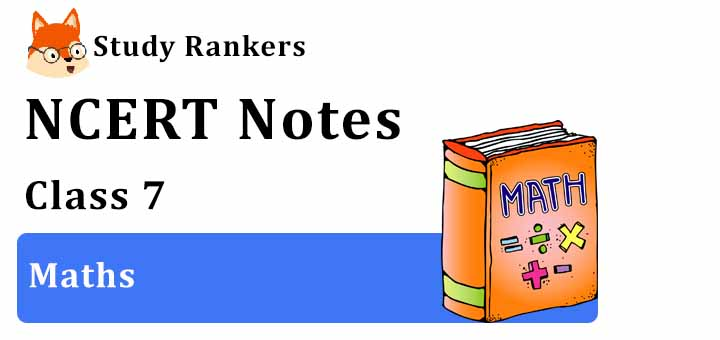Through the help of Revision Notes for Class 7 Maths you can cover plethora of interesting topics in an engaging way. It will be useful in learning about important formulas and points given inside the chapter. You will understand the various factors through which one can improve their efficiency and eventually scores higher in the exam. Along with NCERT Solutions for Class 7 Maths, it is very important guide that will ace up preparations. These Class 7 Revision Notes are quite easy in retaining the answers, once you are fully aware of the concept thus notes can be beneficial for you.

NCERT Class 7 Notes becomes a vital resource for all the students to self-study from NCERT textbooks carefully. You can download PDF of Class 7 Maths Revision Notes that will make entire memorizing process effortless and entertaining. You can view chapterwise Revision Notes for Class 7 Maths by clicking on the chapter name given below.In these NCERT Revision Notes for Class 7, information are arranged in manner that will make learning easier and more understandable. You need to recollect concepts of the chapter in the process of test and tried to provide accordingly. Through these Class 7 Maths notes a student can boost their preparation and assessment of understood concepts.

Chapter 1 Integers

In this chapter, you will learn about important topics such as properties  of addition and subtraction of integers, multiplication and division of integers that will build fundamentals that can be used in higher classes.

Chapter 2 Fractions and Decimals

Chapter 2 Class 7 Maths will help you in studying about multiplication and division of fractions and decimals which will develop your understanding of the topics.

Chapter 3 Data Handling

Data Handling Class 7 Maths will help you in knowing about various terms Arithmetic Mean, Range, Mean and Mode also about bar graphs briefly which are very useful in upcoming classes.

Chapter 4 Simple Equations

In the chapter 4, we will learn about what an equation is and how to solve equation via a systematic method and transposition method which will make you easy to understand various concepts.

Chapter 5 Lines and Angles

Chapter 5 Class 7 Maths notes deals with various types of angles such as complementary, supplementary vertically opposite angles and pair of lines such as parallel lines and transversal line that will broaden your skills.

Chapter 6 The Triangle and Its Properties

In the Chapter 6 Notes for Class 7 Maths we will study about types of triangles based on sides and angles and various properties of triangles such as Property of Exterior Angles, Angle sum property, Pythagoras Property that will clear your doubts.

Chapter 7 Congruence of Triangles

In Chapter 7 Class 7 Maths, as per title we will learn about various types of congruence criterion such as SSS, SAS, ASA and RHS that will develop your logical skills.

Chapter 8 Comparing Quantities

Comparing Quantities Class 7 Maths will let you know various new terms such as ratio and proportion, percentages and finding simple interest that are useful in higher classes.

Chapter 9 Rational Numbers

We will start Chapter 9 by learning about what is rational numbers and then after study about Positive and Negative Rational Numbers and also about operations on rational numbers.

Chapter 10 Practical Geometry

In Chapter 10 Class 7 Maths, we will learn to draw parallel lines and some types of triangles which will be helpful in sharpening your skills.

Chapter 11 Perimeter and Area

Chapter 11 Maths Class 7 consist of various formulas that are useful in finding the area and perimeter of various types of figures such as squares, circles.

Chapter 12 Algebraic Expression

Algebraic expression is formed from variables and constants using different operations. In Chapter 12, we will first start with types of Algebraic Expressions and then after do Addition and Subtraction of these expressions.

Chapter 13 Exponents and Powers

Exponents are used to express large numbers in shorter form. In Chapter 13, we will know about various laws of exponents and use them to solve problems given in the chapter.

Chapter 14 Symmetry

An object is symmetrical when it is the same on both sides. Chapter 14 Class 7 Maths will be helpful in understanding about line symmetry for various polygons, rotational symmetry and mirror reflection.

Chapter 15 Visualising Solid Shapes

In Chapter 15, we will learn about Faces, Vertices and Edges of three dimensional shapes and learn to drawing solids on a flat surface.